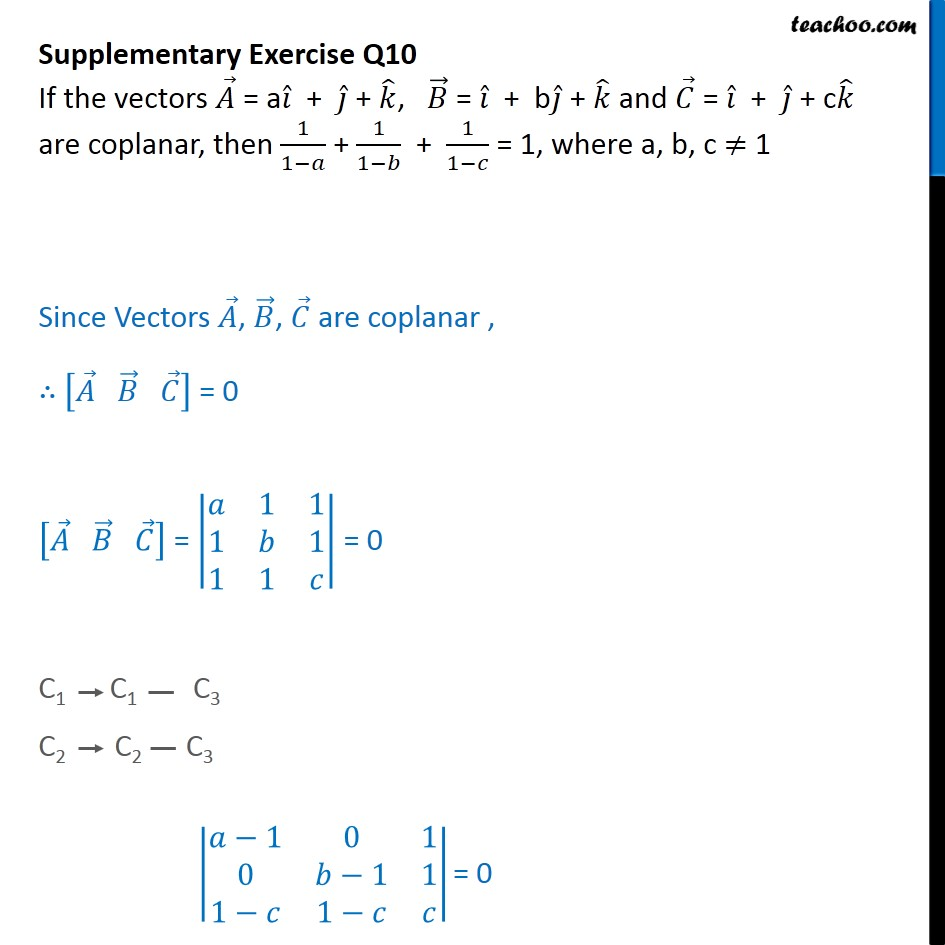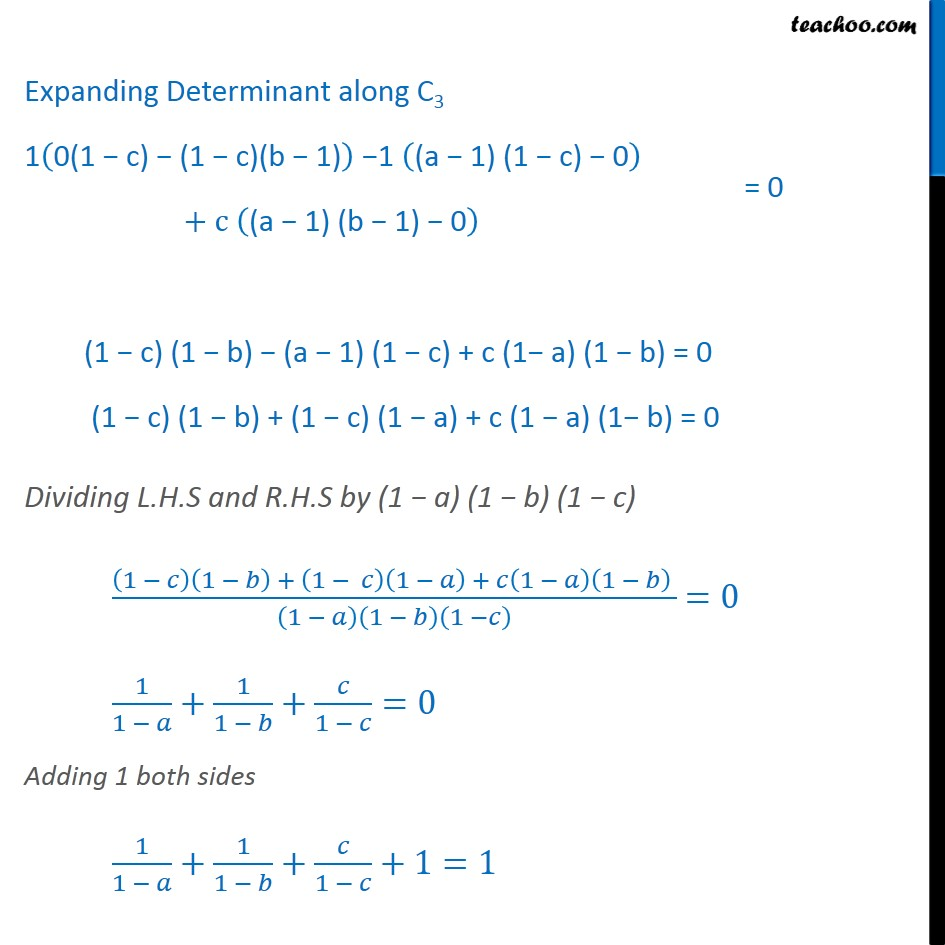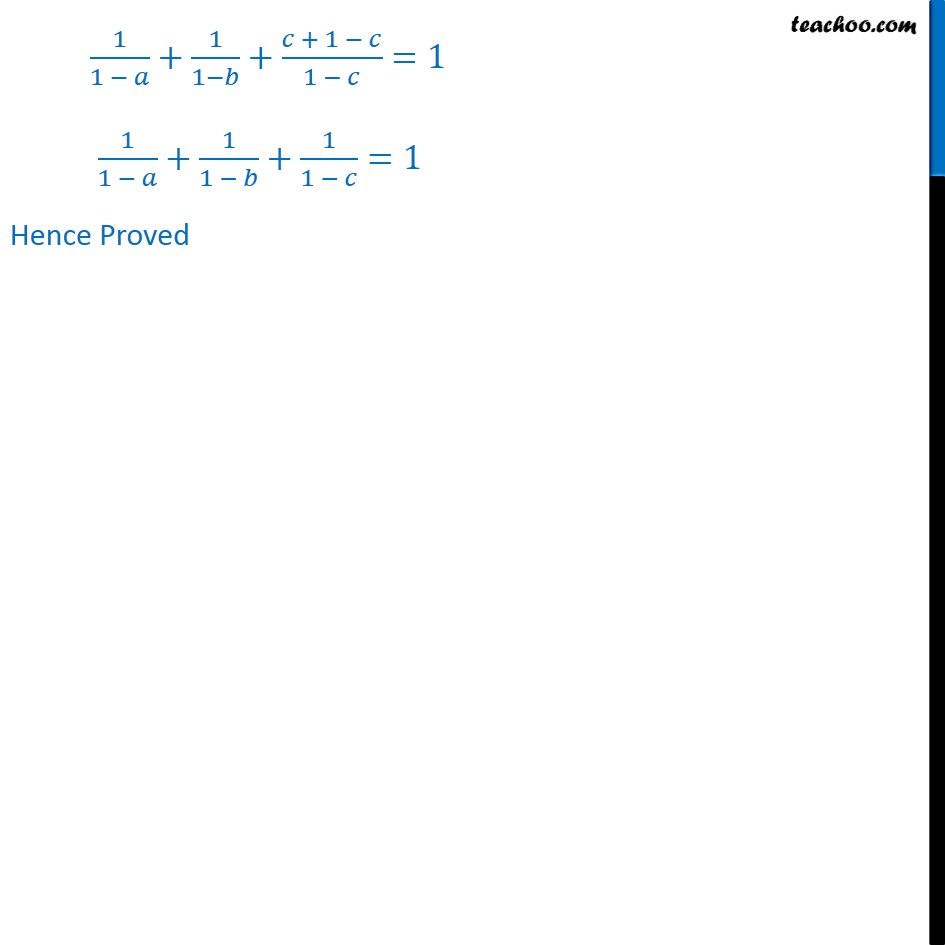Subscribe to our Youtube Channel - https://you.tube/teachoo

1. Chapter 10 Class 12 Vector Algebra
2. Serial order wise
3. Supplementary examples and questions from CBSE

Transcript

Supplementary Exercise Q10 If the vectors = a + + , = + b + and = + + c are coplanar, then 1 1 + 1 1 + 1 1 = 1, where a, b, c 1 Since Vectors , , are coplanar , = 0 = 1 1 1 1 1 1 = 0 C1 C1 C3 C2 C2 C3 1 0 1 0 1 1 1 1 = 0 1 0 1 0 1 1 1 1 = 0 Expanding Determinant along C3 1 0(1 c) (1 c)(b 1) 1 (a 1) (1 c) 0 + c (a 1) (b 1) 0 (1 c) (1 b) (a 1) (1 c) + c (1 a) (1 b) = 0 (1 c) (1 b) + (1 c) (1 a) + c (1 a) (1 b) = 0 Dividing L.H.S and R.H.S by (1 a) (1 b) (1 c) 1 1 + 1 1 + 1 1 1 1 1 =0 1 1 + 1 1 + 1 =0 1 1 + 1 1 + 1 =0 Adding 1 both sides 1 1 + 1 1 + 1 +1=1 1 1 + 1 1 + + 1 1 =1 1 1 + 1 1 + 1 1 =1 Hence Proved

Supplementary examples and questions from CBSE